#### You may also like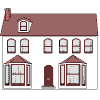### Homes

Six new homes are being built! They can be detached, semi-detached or terraced houses. How many different combinations of these can you find?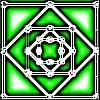### Number Squares

Start with four numbers at the corners of a square and put the total of two corners in the middle of that side. Keep going... Can you estimate what the size of the last four numbers will be?### I'm Eight

Find a great variety of ways of asking questions which make 8.

# What's in a Name?

##### Age 5 to 7Challenge Level
Have a look at these two squares of numbers: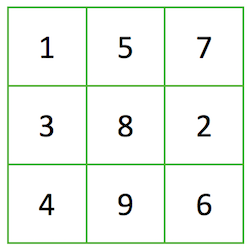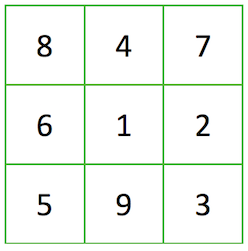What do you see?

What is the same about the two squares?  What is different?

'Magic' squares are square grids with a special arrangement of numbers in them. The arrangement is special because the numbers in each row, column and diagonal add up to the same total. (We could call this the 'magic total'.)

Looking at the left hand square above, if we add the top row of numbers, 1 + 5 + 7, we get a total of 13: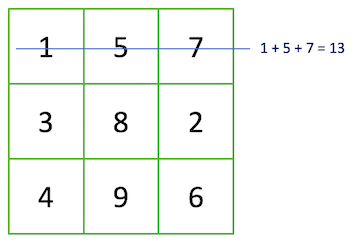If we add the left hand column of numbers, 1 + 3 + 4, we get a total of 8.
So, we know already that this square is not a magic square as these two totals are different.

Use the numbers 1 to 9 to create a magic square.
Can you find another way of doing it?
And another? And another? ...